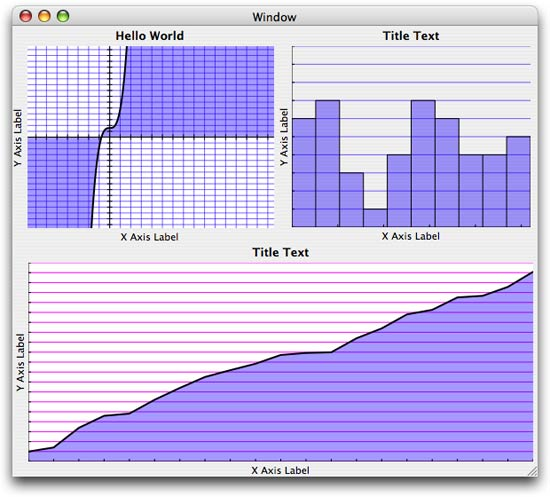# GraphX Plugin

The recent FaceSpan 5.0d42 build includes a graphing plugin based on Chad Weider’s GraphX framework. Chad’s framework provides three graphing views: curve, histogram and scatter plot.

As always when creating FaceSpan plugins from Cocoa frameworks, the tough part is converting the Cocoa APIs into a simple to use AppleScript syntax. In the case of these graphing views, I have substituted the ‘Data Source’ based APIs with a simple event for the curve view, and a value property that accepts a list of values to be graphed (a list of reals for the histogram and a list of x-y points for the scatter plot).

Here’s how it all looks running in a FaceSpan-built application:The code for the curve view looks like this:

```--
--  CT curve uses this event handler to get the data to be graphed
--
on CT get y value theCTCurve for theXValue
return theXValue * theXValue * theXValue + 1.0
end CT get y value
```

The event is called repeatedly by the curve view to generate the data to be graphed.

The code for the histogram looks like this:

```on initialize theResponder
--  CT histogram needs a list of reals to graph
set theData to {}

repeat with i from (x axis minimim) to (x axis maximum)
set end of theData to random number from 1 to 10
end repeat
set value to theData

end initialize
```

Here I simply generate a random series of values and assign them to the view’s value property. The plugin does the rest.

And finally, the code for the scatter plot looks like this:

```on initialize theResponder
--  CT scatter plot requires a list of points (x, y pairs) to graph...

set yValue to 1.0
set theData to {}

repeat with i from (x axis minimim) to (x axis maximum)
set end of theData to {i, yValue}
set yValue to yValue + (random number from 0 to 2.0)
end repeat
set value to theData

end initialize
```

Again, I simply generate a random series of point values and assign them to the view’s value property. The plugin does the rest.

The sources for the plugin are included in the FaceSpan 5.0d42 SDK.